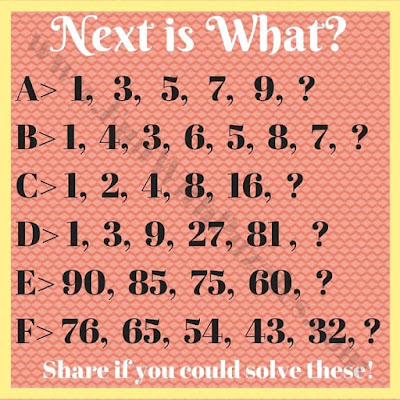In number sequence puzzles one has to find the next number in the given series of numbers. In these puzzles, some numbers are given and these numbers follow some logical or mathematical patterns. In these puzzles, your challenge is to find the logical or mathematical pattern in these given numbers and have to find the next number in the sequence.
These Find the next number in the sequence puzzles are very easy brain teasers and all these puzzles are for kids. These are the basic puzzles for someone who is the first time doing these puzzles to give the introduction to these puzzles. Later we will be posting some tough puzzles in this category.
Answers to these puzzles will be provided at the end of this post.1. Can you find the next number in these puzzles?

Do checkout below listed Maths Puzzles and Brain Teasers to enhance your brainpower
List of Maths Puzzles and Brain Teasers

1. Mind Twisting Math Pattern Series Riddles with answers: These are the tough Maths pattern series puzzles in which your challenge is to find the next number in the series.

2. Pattern-Find the next image in the sequence?: These are similar to find the next number in the sequence. However, in these Picture Puzzles, your challenge is to find the next image in the sequence of images.

3. Math Circle Brain Teasers with Answers for Teens & Adults: These are the Mathematical Brain Teasers in which your challenge is to find the missing number in the Circle.

4. Missing number puzzles with answers: Another set of Maths Picture Puzzles in which your task is to find the missing number which will replace the question mark.

A. 11. This is the sequence of Odd Numbers.

B.  10. There are two series in this sequence. One is the Odd Numbers series and another is the Even number series.

C.  32. The number is getting doubled in each term as compared to the previous term.

D. 243. The previous term is multiplied by the number 3.

E. 40. The difference in each term is getting increased by 5 as compared to the previous difference.

F. 21. The difference between each term is 11.